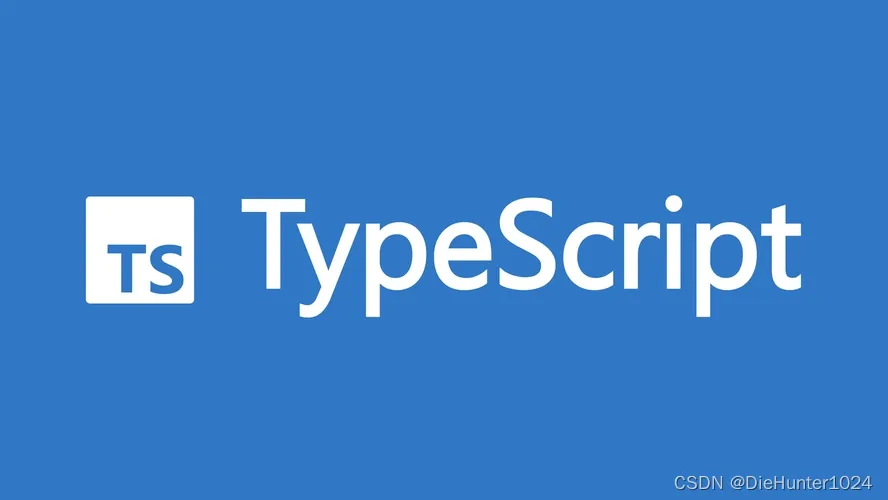# TypeScript（三）对象类型

Object与object有什么区别？

Array（数组）

Function（函数）

Tuple（元组）

Enum（枚举）

计算类型：

Object（对象）# 对象类型

## Object与object有什么区别？

object是TypeScript中的类型（在JS中不存在），它是非基本数据类型的统称，包括普通对象、数组、函数等等

const obj: Object = 1 // 正常运行
const obj1: object = 1 // error 不能将类型“number”分配给类型“object”

const obj: object = {} // 对象
const arr: object = [1, 2, 3] // 数组
const fn: object = function () { // 函数
console.log(obj);
}
const tuple: object = ['count', 10] // 元组
const map: object = new Map() // 键值对集合
const set: object = new Set() // 无序集合

const obj: Object = {}
const arr: number[] = [1, 2, 3]
const fn: Function = function () {
console.log(obj);
}
const tuple: [string, number] = ['count', 10]
const map: Map<number, string> = new Map()
const set: Set<string> = new Set()
obj.valueOf()
arr.push(4)
fn()
tuple.push(1)
map.set(0, "0")
set.add('hello')

## Array（数组）

const arr: string[] = ['a', 'b']
const arr3: string[][] = [['a'], ['b']] // 二维数组

const arr1: Array<number> = [1, 2]
const arr2: Array<Array<number>> = [[1, 3], [1, 5]] // 二维数据

const arr: string[] = ['a', 'b'] // 方式一
interface IArray {
[i: number]: string
}
const arr2: IArray = arr// 方式二

## Function（函数）

const fn: Function = function (a: number, b: number) {
return a + b
}

const fn: Function = function (a: number, b: number): number {
return a + b
}

const fn: Function = (a: number, b: number): number => {
return a + b
}

此外，使用另一种函数定义写法可以具象表示函数的参数以及返回值

let fn: (a: number, b: number) => number = null
fn = (a, b) => {
return a + b
}

let fn: () => void = null
fn = () => { }

## Tuple（元组）

const tuple: [number, string] = [0, "张三"]
tuple = 1
tuple = "李四"

const tuple: [number, string] = [0, "张三"]
tuple.push(1) // 不抛错
tuple.push(false) // 抛错，提示boolean类型不属于number和string

## Enum（枚举）

enum Color {
red,
blue,
yellow
}
console.log(Color);// { '0': 'red', '1': 'blue', '2': 'yellow', red: 0, blue: 1, yellow: 2 }

var Color;
(function (Color) {
Color[Color["red"] = 0] = "red";
Color[Color["blue"] = 1] = "blue";
Color[Color["yellow"] = 2] = "yellow";
})(Color || (Color = {}));
console.log(Color); // { '0': 'red', '1': 'blue', '2': 'yellow', red: 0, blue: 1, yellow: 2 }

### 数字：

enum Color {
red = 3,
blue = 5,
yellow = 7
}
console.log(Color);// { '3': 'red', '5': 'blue', '7': 'yellow', red: 3, blue: 5, yellow: 7 }

### 字符串：

enum Color {
red = "1",
blue = "2",
yellow = "3"
}
console.log(Color);// { red: '1', blue: '2', yellow: '3' }

### 计算类型：

enum Color {
red = 1,
blue = red + 1,
yellow = blue + 1
}
console.log(Color);// { '1': 'red', '2': 'blue', '3': 'yellow', red: 1, blue: 2, yellow: 3 }

### 二进制计算：

enum Direction {
Up = 0b1000,
Down = Up >>> 1,
Left = Down >>> 1,
Right = Left >>> 1,
}
console.log(Direction);
/*{
'1': 'Right',
'2': 'Left',
'4': 'Down',
'8': 'Up',
Up: 8,
Down: 4,
Left: 2,
Right: 1
}*/

enum Direction {
Up = 0b1000,
Down = Up >>> 1,
Left = Up >>> 2,
Right = Up >>> 3,
}

### 异构枚举（不同类型的初始值）：

enum Result {
Fail = 0,
Success = "success",
}

## Object（对象）

const obj: Object = {
name: "张三",
age: 20
}

console.log(obj);// { name: '张三', age: 20 }

# 总结

TypeScript 入门教程

TypeScript: Documentation - 基础

posted @ 2023-03-05 14:45  阿宇的编程之旅  阅读(108)  评论(0编辑  收藏  举报